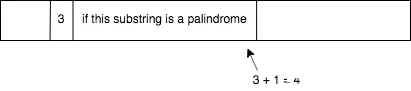# Minimum Palindrome Partitions

Posted on:December 14, 2019

A cut is defined as a slice of a string that partitions a string into two. Given a string, return the minimum number of cuts required to partition the string into palindromic substrings.

Some examples:

`a` would return 0, since `a` is already a palindrome and no cuts are required.

`asa` would also return 0, since `asa` is also a palindrome.

`as` would return 1, since one cut is required: `a|s`.

`aasaadl` would return 2: `aasaa|d|l`.

The solution is a brilliant usage of dynamic programming - one of those times where you’re completely stuck at first but seems so obvious once you know it.

What we’re memoizing is the number of cuts required for each of the prefixes, and running mid-point checks from each of the starting points (not forgetting to take both odd and even length palindromes into account). Running time is quadratic in the length of the string, and space required is linear.As you can see, when we detect a palindrome, we update the `cuts` array with a previous value of `cuts` (which we already know to be optimal) + 1.

``````def minimum_palindrome_partitions(s):
# cuts[i] = how many palindrome partitions required to cut s[:i]
# we initialize it as i-1 first since that's the maximum number of cuts possibly required (if all characters are distinct)
cuts = [i-1 for i in range(len(s) + 1)]
# check each center of palindrome candidate
for i in range(len(s)):
# at each center, check odd length palindromes
for j in range(min(i + 1, len(s) - i)):
if s[i - j] == s[i + j]:
cuts[i + j + 1] = min(cuts[i + j + 1], cuts[i - j] + 1)
else:
break
# at each center, check even length palindromes
for j in range(min(i, len(s) - i)):
if s[i - j - 1] == s[i + j]:
cuts[i + j + 1] = min(cuts[i + j + 1], cuts[i - j - 1] + 1)
else:
break
return cuts[-1]``````